# Using 3D Hidden Markov Models that explicitly represent spatial coordinates to model and compare protein structures

## Abstract

### Background

Hidden Markov Models (HMMs) have proven very useful in computational biology for such applications as sequence pattern matching, gene-finding, and structure prediction. Thus far, however, they have been confined to representing 1D sequence (or the aspects of structure that could be represented by character strings).

### Results

We develop an HMM formalism that explicitly uses 3D coordinates in its match states. The match states are modeled by 3D Gaussian distributions centered on the mean coordinate position of each alpha carbon in a large structural alignment. The transition probabilities depend on the spread of the neighboring match states and on the number of gaps found in the structural alignment. We also develop methods for aligning query structures against 3D HMMs and scoring the result probabilistically. For 1D HMMs these tasks are accomplished by the Viterbi and forward algorithms. However, these will not work in unmodified form for the 3D problem, due to non-local quality of structural alignment, so we develop extensions of these algorithms for the 3D case. Several applications of 3D HMMs for protein structure classification are reported. A good separation of scores for different fold families suggests that the described construct is quite useful for protein structure analysis.

### Conclusion

We have created a rigorous 3D HMM representation for protein structures and implemented a complete set of routines for building 3D HMMs in C and Perl. The code is freely available from http://www.molmovdb.org/geometry/3dHMM, and at this site we also have a simple prototype server to demonstrate the features of the described approach.

## Background

HMMs have been enormously useful in computational biology. However, they have only been used to represent sequence data up to now. The goal of the present work is to make HMMs operate fundamentally with 3D-structural rather than 1D-sequence data. Since HMMs have proven worthwhile in determining a characteristic profile for an ensemble of related sequences, we expect them to be useful in building a rigorous mathematical description of protein fold family. Our work rests on three elements of background theory: 1D HMMs, 3D structural alignment and 3D core structures.

### One-dimensional HMMs

Profile hidden Markov models (profile HMMs) are statistical models of the primary structure consensus of a sequence family. Krogh et al  introduced profile HMMs to computational biology to analyze amino acid sequence similarities, adopting HMM techniques that had been used for years in speech recognition . This paper had a propelling impact, because HMM principles appeared to be well suited to elaborating upon the already popular "profile" methods for searching databases using multiple alignments instead of single query sequences . In this context an important property of HMMs is their ability to capture information about the degree of conservation at various positions in an alignment and the varying degree to which indels are permitted. This explains why HMMs can detect considerably more homologues compared to simple pairwise comparison [4, 5]. Since their initial use in modeling sequence consensus, HMMs have been adopted as the underlying formalism in a variety of analyses. In particular, they have been used for building the Pfam database of protein familes , for gene finding , for predicting secondary structure  and transmembrane helices . Efforts to use sequence-based HMMs for protein structure prediction , fold/topology recognition  and building structural signatures of structural folds  were also reported recently. However, no one yet has built an HMM that explicitly represents a protein in terms of 3D coordinates. A further key advantage of using HMMs is that they have a formal probabilistic basis. Bayesian theory unambiguously determines how all the probability (scoring) parameters are set, and as a consequence, HMMs have a consistent theory behind gap penalties, unlike profiles.

A typical HMM (see Figure 1) consists of a series of states for modeling an alignment: match states M k for consensus positions; and insert I k and delete states D k for modeling insertions/deletions relative to the consensus. Arrows indicate state-to-state transitions, which may occur according to the corresponding transition probabilities. Sequences of states are generated by the HMM by following a path through the model according to the following rules:

• The path is initiated at a begin state M0; subsequent states are visited linearly from left to right. When a state is visited, a symbol is output according to the emission probability of that state. The next state is visited according to current state's transition probabilities.

• The probability of the path is the product of probabilities of the edges traversed. Since the resulting sequence of states is observed and underlying path is not, the part of the HMM considered "hidden" is the path taken through the model.

### Structural alignment

Structural alignment involves finding equivalences between sequential positions in two proteins (Figure 2). As such, it is similar to sequence alignment. However, equivalence is determined on the basis of a residue's 3D coordinates, rather than its amino acid "type." A number of procedures for automatic structural alignment have been developed . Some of these are based on iterative applications of dynamic programming, where each iteration minimizes the RMS distances between the newly aligned atoms. Others maximize the overlap of distance matrices, and yet others are based on heuristics such as hashes. Structural alignment has been used to find non-obvious similarities in protein structure – e.g. the globin-colicin similarity  – to cluster the whole structure databank , to refine measures of structural annotation transfer , and to assist homology modeling .

The next step after pairwise structural alignment is multiple structural alignment, simultaneously aligning three or more structures together. There are currently a number of approaches for this [18, 23, 31, 32]. Most of these proceed by analogy to multiple sequence alignment , building up an alignment by adding one structure at a time to the growing consensus. Multiple structural alignment is an essential first step in the construction of consensus structural templates, which aim to encapsulate the information in a family of structures. It can also form the nucleus for a large multiple sequence alignment – i.e., highly homologous sequences can be aligned to each structure in the multiple alignment.

### Core structures

Given a structural alignment of a family of proteins, a set of atoms with essentially fixed relative positions in all member of the family can be determined [36, 37]. This set is called the invariant core for the given family. Cores are meant to characterize protein families statistically, based on positional variation in observed main chain atoms among members of structural family.

Computing a core structure involves the following steps: Superimposition (structural alignment) of an ensemble of protein structures from the same family, calculation of structural deviation between coordinates from ensemble structures, iterative removal of non-core atoms based on high positional variation, calculation of ellipsoid volumes representing positional variance of alpha carbon positions at every core center.

### Difficulty in adapting 1D HMM Formalism to 3D

There have been a number of recent attempts to make HMMs be useful for structural studies [9, 10]. However, none of the suggested schemes are fundamentally three-dimensional (coordinate dependent), since all of them are based on building a 1D HMM profile representing a sequence alignment and structural information only enters in the form of encoded symbols (i.e. H for helix and E for sheet). Adding in real 3D structure is non-trivial. This reflects the fact that the structure is fundamentally different from the sequence not only in increased dimensionality, but also due to the transition from discrete to continuous representation. For matching a query structure of the model, the conventional dynamic programming that underlies normal HMMs will not work since structural alignment is "non-local". Normal dynamic programming assumes that determining the best match between query and model in a given "local" region of the alignment potentially will not affect the optimum match sequentially before this region. Thus, one can break up the whole sequence comparison problem into sub-problems that can be readily solved. However, this does not apply in structure comparison. The optimum match in one region of the alignment R potentially can affect the optimum superposition between two structures and this in turn can affect the optimum alignment globally, in regions sequentially distant from R.

### Combining 1D HMMs with 3D Cores to construct 3D HMMs

To get around this difficulty, we develop a way of doing an alignment-free superposition initially and then adding conventional HMM scoring. In our approach, the core structures are made from "ellipsoidal" Gaussian distributions centered on aligned Cα positions. If each Gaussian distribution is normalized to 1, we have a probability distribution based on coordinates. Hence, if we want to use HMMs on protein structures instead of sequences, core models provide a nice representation for the match states. In fact, we can think of the cores as "structural profiles", with each core ellipsoid representing a statistical distribution of potential coordinates, just as in sequence profiles, each match state represents a probability distribution of each of the 20 amino acids occurring in that position. In practice for a sequence profile, state emission distributions correspond to tables of probabilities of each amino acid appearing. Likewise, in 3D HMMs match states correspond to the probability of a given Cα position falling within a prescribed volume. This can be readily calculated from coordinate differences: if an aligned Cα from the query appears close to the ellipsoid centroid, it scores well; if it is farther away, it scores poorly.

A path through a HMM is a sequence of states such that there exists an edge from each state in the path to the next state in the path. A path through the 3D HMM gives a probability distribution of each structural position, based on the probabilities of the atom positions in the corresponding states. The probability of the structure given a core target is the product of the probabilities of the Cα positions in each state. The probability of an HMM generating a structure is the sum, over all the paths in the HMM, of the probability of the path times the probability of the structure given the path.

Transition probabilities of the new model are based on the probabilities of the coordinates of a given atom within a coordinate distribution (ellipsoid), where the probabilities are calculated based on the relative distance of the query atom from each ellipsoid centroid. For regions not associated with the core transition probabilities are derived from a multiple alignment in a similar fashion to those in 1D sequence HMMs, and we employ a Bayesian approach to merge them together.

We, therefore, suggest a combined approach. A separate HMM is computed for each core structure we want to use. Every core ellipsoid corresponds to the match state in the HMM. The sizes of the ellipsoids are related to the HMM transition probabilities: the larger size corresponds to a greater indel probability, whereas the smaller one indicates a more conserved core position and thus increased probability of transition to the corresponding match state (and a lower probability of falling into an indel state). Next, a query protein structure is aligned with a core and relative interstructural distances are computed. These deviations between the query and the core model can be converted to the emission probabilities in the match states of the HMM, and an overall score can finally be computed. This score represents the log likelihood that the protein structure was generated by the HMM, and not some other disordered model, called the null model. In practical terms, this score can be used to identify the new protein as a potential member of a protein family, and thus classify it.

## Results and Discussion

To demonstrate the power of our three-dimensional probabilistic constructs for protein structure analysis, we performed the following calculations. First, we built 3D HMMs for globin and IgV fold families from the sets of aligned representative structures. Eighteen structures were chosen to create a 3D HMM for the globin family and four structures to represent the IgV domains. We selected a small number of representatives for the IgV HMM in an attempt to establish a statistically meaningful threshold for the minimal size of a typical training set. We scored all available globin and IgV SCOP domains against our constructed models. The separation of scores appeared to be quite good (Figure 4). Globin 3D HMM and IgV 3D HMM turned out to be capable of distinguishing the queries from its own class with 98.2% and 96.6% accuracy, respectively. We explain minor overlaps of the scores by incompleteness of the chosen training sets as well as by our inability to achieve optimal superposition at the structural alignment stage for certain cases. To the contrary, the corresponding RMSD values calculated for the alignment of all IgV's and globin domains against the IgV core (match states from the IgV 3D HMM) resulted in quite significant overlap and appeared to be much less informative for structural classification (Figure 5). We use RMSD values as a benchmark because the procedure involving structural alignment of the query against the core remains the same in both RMSD and 3D HMM calculations, whereas the improvement of the 3D HMM scores separation comes exclusively from the HMM scoring scheme.

To further test performance of our method we performed more challenging, (α/β) vs (α/β) and (α+β) vs (α+β) classification-type calculations: FAD/NAD(P)-binding domains (SCOP fold 51904) vs NAD(P)-binding domains (SCOP fold 51734), Flavodoxin fold (SCOP fold #52171) vs Thioredoxin fold (SCOP fold #52832) and Ferrodoxin fold (SCOP fold #54861) vs Lysozyme fold (SCOP fold #53954). Each model was built by choosing from five to ten non-identical (less than 95% sequence identical) representatives, which had RMSD no greater than 3 Angstroms (within the set) and the minimal available length of sequence consensus. Insert-insert transition probabilities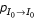and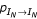were manually set equal to 0.95 in order to minimize the insertion penalties at the terminal ends of the model (to model the flanking ends of the longer structures which didn't enter the training set). Thus, our "minimal core" models effectively scored the best superimposed part of the query with respect to the core centroids. Note, that in the corresponding RMSD calculation, the flunking ends do not affect the RMSD value, because only the distances for the determined query ↔ model equivalence pairs enter the score. Overlap of the 3D HMM log scores for these calculations never exceeded 15% in the calculations (Figures 6,7,8), whereas the RMSD scores overlapped more than 65% (not shown).

We also scored all 95% or less sequence-identical domains against the 3D HMM for Thioredoxin fold (Figure 9). One can readily see that the scores for all non-Thioredoxin folds are located mostly to the right of the scores typical for the Thioredoxin fold.

Finally, we performed a different classification-type calculation: given a 3D HMM built for the ferrodoxin family from four representative structures, we scored the same globin and IgV domains against it. Even though none of the queries belonged to the ferrodoxin 3D HMM, the separation of HMM scores for the two folds was still as high as in the previous calculations, namely 97.5%. This suggests that a given model may be useful not only for binary classification but also for dividing up fold space in more distance oriented terms. On the other hand, the corresponding RMSD values ranged from 4 to 11 Angstroms with no detectable separation at all. This can be readily understood: a 3D HMM is designed in such a way that different spatial regions are assigned different spatial penalties in the form of the coordinate-dependent emission, insertion and deletion probabilities. Therefore, when aligned with the core of the ferrodoxin HMM, queries from different families would encounter different and very specific for each family score penalties for appearing in the "wrong", family-specific regions of space. RMSD values for such alignments can be almost identical for the two families; however, the corresponding HMM scores will, most probably, appear confined within relatively small and, in general, separable intervals. In contrast, two queries, one with seemingly large RMSD score and another one with a much smaller value, may still rightfully belong to the same 3D HMM class, provided that the first query has its high RMSD structural parts appearing in the "allowed" (low-penalty) spatial regions. With help of this highly useful 3D HMM feature, one should be able to develop a scale of scores for various structural groups of interest, which can be further used for classification of the unknown structures (or parts of structures) in the same manner as chemical shifts for different functional groups are used in NMR structure determination experiments.

## Conclusion and future directions

We have created a rigorous three-dimensional HMM representation for protein structures. This incorporates the major elements of HMM theory: a set of directionally connected match, insert, and delete states, transition and emission probabilities, the optimal Viterbi path, and forward algorithm. We have not yet considered such aspects as using Expectation maximization and the Baum-Welch procedure for optimization of model parameters. However, currently our procedure is self-contained and ready for protein structure analysis. Preliminary results indicate that the new constructs can be quite useful for protein structure analysis and classification.

## Methods

In outline our procedure consists of the following steps:

1. 3D representation for the match states and emission probabilities;

2. A procedure for superposing a query against the match states without consideration of transitions;

3. Calculation of transition probabilities from the core parameters and evaluation of corrections from observed gaps in a multiple alignment;

4. Modified forward and Viterbi algorithms for calculating the probability that the query was generated from the 3D HMM and calculating the best alignment of the query to the model.

A conventional 1D HMM would comprise steps 3 and 4. However, because we are dealing with 3D coordinates a single application of the dynamic programming in the Viterbi sense is not sufficient to match a query against the model. The reason is that changing locally the match between query and model affects the global overall superposition of the structure. Hence, we have to find a superposition first in heuristic fashion without consideration of the transitions before scoring with transitions. To achieve this optimal superposition, we align the centers of the ellipsoids (match states in the model) with the Cα coordinates of the query.

### Three-dimensional core representation for HMM match states

The parametric representation of the core structure is built by modeling a distribution of atomic positions as a 3D Gaussian. Having a set of structures, superimposed using an RMS criteria, we obtain an alignment, which pairs each atom k in one structure with an equivalent atom in the others. The mean position r k ≡ (x k ,y k ,z k ) and 3D-covariance matrix for each such center k can be calculated: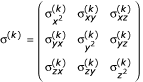We can now use this statistical representation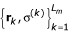to position a query structure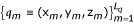with respect to the centers of the obtained core structure. The modified relative distances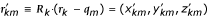of the m-th query atom with respect to the k-th core position are obtained by applying the rotation matrix R k , which diagonalizes the covariance matrix σ(k),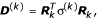where D(k)is a diagonal matrix whose diagonal elements d(k)are the variances of a 3D distribution of the k-th ellipsoid that is oriented along the global coordinates. Clearly, core ellipsoids represent the emission probability distributions for the match states, i.e. the probability that a query atom is emitted by the match state located at the center of the corresponding ellipsoid.

Since the protein backbone is not continuous we have to discretize our emission probability distributions in order to obtain finite values for the observed (emitted), finite series of the query atoms. In the present work we choose variances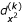,and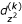to make this discretization. Figure 3 illustrates discretization of a Gaussian distribution in one-dimensional case. Each distribution is partitioned amongst intervals equal to the corresponding variance value, i. e.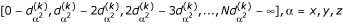. The values of the distribution integrated over each interval give the desired values for the discretized emission probability distribution: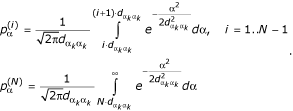In practical computer implementation the integrals are replaced by numerical values of the gamma function Γ (a) and incomplete gamma function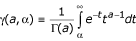by using relationship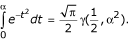Note that our discretization procedure effectively partitions the volume of the k-th ellipsoid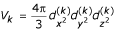, the measure of the penalty for the deviation from the ellipsoid center, creating a discrete representation of the emission probability distribution for each match state k. The observed emission probabilities (scores) e kn – i.e. those resulting from the alignment of the query with the core – will be given as a product of the corresponding discretized emission probabilities for each coordinate: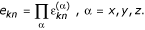The component emission probabilities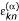are determined from following relation: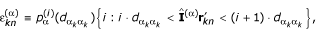where operatorprojects out component α from vector.

### Calculation of transition probabilities from core parameters and evaluation of corrections from alignment gaps

In addition to setting the values of the match emission values, volumes of the core ellipsoids can help parameterize the values for the transitions. A larger ellipsoid volume is associated with a greater chance of having an indel nearby, thus decreasing the probability of going into the corresponding match state. Therefore, it is logical to assume that the probability of transition to the match state should be inversely proportional to the volume of the corresponding core ellipsoid. In the present work we suggest the following values for priors on the transition probabilities: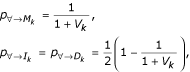wheredenotes any state in the model from where transition is allowed by the inherent HMM topology. One can easily see that the above formulas produce higher penalties for transitions deviating from highly conserved core positions (match states).

We use the observed pattern of gaps in a known multiple sequence alignment as corrections to the above formulas for the transition probabilities. In particular, gaps in the sequence alignment corresponding to the non-core regions designate spaces with the higher insertion probabilities, whereas missing atoms in each core position is a sign of increasing deletion probability. The sequence alignment corrections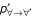(representing any of the I k , D k or M k states) can be introduced by direct application of pseudocount technique, which is easily formalized in Bayesian framework :where M is the number of structures in the (training) multiple alignment;is the total number of amino acid symbol absences within a gap immediately preceding a particular HMM match state k,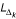is the length of the gap, and n0 is a so-called pseudocount constant , which scales the correction. In the above formula,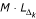represents the total number of symbols within the gap (counting both amino acid symbols and amino acid absence symbols), and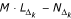gives the number of amino acid symbols present in the gap. If the pseudocount constant n0 is set equal to 1, the above formula transforms to a well-known Laplace rule .

The corresponding corrections resulting from the gaps observed at actual HMM match states are calculated in the same manner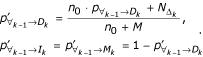The final value for the transition probability is the renormalized sum of the priors and the corresponding corrections: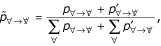where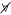denotes any HMM state accessible from state. The optimization of the obtained probabilities with respect to maximization of the scores for the training sets may be necessary for the final tune-up.

### Viterbi and Forward algorithms for aligning and scoring the query

Once one has two structures (the query and the centers of the core ellipsoids) optimally aligned in a gap-independent fashion and has a set of transition probabilities calculated between the match states, one can use standard HMM methods to calculate the single best alignment between the query and the model (the Viterbi algorithm) and the overall score for matching the query to the model, integrated over all possible paths through the model (the forward algorithm). Our implementation of the Viterbi algorithm is straightforward, following that in Durbin et al. . However, we rederived the Forward Algorithm equations  for the case of fully interconnected HMM using matrix calculus notations. This made the computer implementation more straightforward and faster for structures. Our forward algorithm equations assume the following form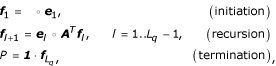where π denotes the initial probability distribution among the possible begin states, A is the transition matrix of dimension L m × L m , e l is the vector of emission probabilities for the state l, f l is the vector of forward variables in the l-th step, 1 is the vector with components all equal to 1, L q is the length of the observation sequence (query), and P is the total probability that the observation sequence was emitted by the given model. Note the distinction between the scalar product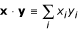and vector multiply operation (Hadamard product)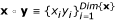that are related as x·y = 1·(x ◦ y).

For the bigger HMMs with number of states 100 and higher, direct application of the above formulas involves many multiplications of small numbers (transition and emission probabilities) and usually results in severe underflow problems in practical computer implementations. These can be avoided if one works with logarithms of the involved quantities, even when one needs to perform addition or subtraction. Furthermore, because of the directional nature of the HMM topology many transition probabilities (those between non-adjacent states) are identically zero. Therefore, instead of dealing with the full L m × L m transition matrix A we can work with three 3 × L m submatrices a M , a D and a I , which represent transitions from the match, delete and insert states respectively. The formulas for the HMM scoring based on the forward algorithm then take the form (with notation identical to that used in ):

Initialization: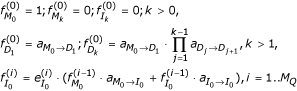Recursion: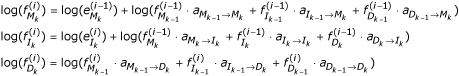Termination: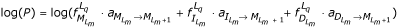Our initialization ofis different from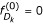, which is given in  for a similar HMM profile. A detailed derivation of the correct initialization condition along with accompanying examples will be published elsewhere.

## References

1. Krogh A, Brown M, Mian IS, Sjolander K, Haussler D: Hidden Markov models in computational biology. Applications to protein modeling. J Mol Biol 1994, 235: 1501–1531. 10.1006/jmbi.1994.1104

2. Rabiner LR: A Tutorial on Hidden Markov Models and Selected Applications in Speech Recognition. Proceedings of the IEEE 1989, 77: 257–285. 10.1109/5.18626

3. Gribskov M, Lüthy R, Eisenberg D: Profile Analysis. Meth. Enz. 1990, 183: 146–159.

4. Teichmann S, Park J, Chothia C: Structural assignments to the proteins of Mycoplasma genitalium show that they have been formed by extensive gene duplications and domain rearrangements. Proc. Natl. Acad. Sci. 1998, 95: 14658–14663. 10.1073/pnas.95.25.14658

5. Reese MG, Kulp D, Tammana H, Haussler D: Genie--gene finding in Drosophila melanogaster [see comments]. Genome Res 2000, 10: 529–538. 10.1101/gr.10.4.529

6. Eddy SR: Hidden Markov models. Curr. Opin. Struc. Biol. 1996, 6: 361–365. 10.1016/S0959-440X(96)80056-X

7. Durbin R, Eddy S, Krogh A, Mitchison G: Biological sequence analysis : probalistic models of proteins and nucleic acids. Cambridge, UK New York, Cambridge University Press 1998, 356.

8. Bateman A, Birney E, Durbin R, Eddy SR, Howe KL, Sonnhammer EL: The Pfam protein families database. Nucleic Acids Research 2000, 28: 263–666. 10.1093/nar/28.1.263

9. Bystroff C, Thorsson V, Baker D: HMMSTR: a Hidden Markov Model for Local Sequence-Structure Correlations in Proteins. Journal of Molecular Biology 2000, 301: 173–190. 10.1006/jmbi.2000.3837

10. Sonnhammer E.L., von Heijne, G., Krogh, A.: A hidden Markov model for predicting transmembrane helices in protein sequences. Intelligent Systems in Molecular Biology 1998, 6: 175–182.

11. Karplus K, Sjolander K, Barrett C, Cline M, Haussler D, Hughey R, Holm L, Sander C: Predicting protein structure using hidden Markov models. Proteins 1997, Suppl 1: 134–139. Publisher Full Text 10.1002/(SICI)1097-0134(1997)1+<134::AID-PROT18>3.3.CO;2-Q

12. Di Francesco V, Garnier J, Munson PJ: Protein topology recognition from secondary structure sequences: application of the hidden Markov models to the alpha class proteins. J Mol Biol 1997, 267: 446–463. 10.1006/jmbi.1996.0874

13. Di Francesco V, Geetha V, Garnier J, Munson PJ: Fold recognition using predicted secondary structure sequences and hidden Markov models of protein folds. Proteins 1997, Suppl 1: 123–128. Publisher Full Text 10.1002/(SICI)1097-0134(1997)1+<123::AID-PROT16>3.3.CO;2-#

14. Di Francesco V, McQueen P, Garnier J, Munson PJ: Incorporating global information into secondary structure prediction with hidden Markov models of protein folds. Proc Int Conf Intell Syst Mol Biol 1997, 5: 100–103.

15. Kersting K, Raiko T, Kramer S, De Raedt L: Towards discovering structural signatures of protein folds based on logical hidden Markov models. Pac Symp Biocomput 2003, 192–203.

16. Taylor WR, Orengo CA: Protein Structure Alignment. J. Mol. Biol. 1989, 208: 1–22.

17. Holm L, Sander C: Protein Structure Comparison by Alignment of Distance Matrices. J. Mol. Biol. 1993, 233: 123–128. 10.1006/jmbi.1993.1489

18. Sali A, Blundell TL: The definition of general topological equivalence in protein structures: A procedure involving comparison of properties and relationships through simulated annealing and dynamic programming. J. Mol. Biol. 1990, 212: 403–428.

19. Godzik A, Skolnick J: Flexible algorithm for direct multiple alignment of protein structures and sequences. CABIOS 1994, 10: 587–596.

20. Artymiuk PJ, Mitchell EM, Rice DW, Willett P: Searching Techniques for Databases of Protein Structures. J. Inform. Sci. 1989, 15: 287–298.

21. Gerstein M, Levitt M: Using Iterative Dynamic Programming to Obtain Accurate Pairwise and Multiple Alignments of Protein Structures. Proc. Fourth Int. Conf. on Intell. Sys. Mol. Biol. Menlo Park, CA, AAAI Press 1996, June 11–15: 59–67.

22. Gerstein M, Levitt M: Comprehensive Assessment of Automatic Structural Alignment against a Manual Standard, the Scop Classification of Proteins. Protein Science 1998, 7: 445–456.

23. Levitt M, Gerstein M: A Unified Statistical Framework for Sequence Comparison and Structure Comparison. Proceedings of the National Academy of Sciences USA 1998, 95: 5913–5920. 10.1073/pnas.95.11.5913

24. Singh A. P., Brutlag, D. L.: Hierarchical Protein Structure Superposition using both Secondary Structure and Atomic Representations. Proc. Intelligent Systems for Molecular Biology 1997, 5: 284–293.

25. Holm L, Sander C: Structural alignment of globins, phycocyanins and colicin A. FEBS Lett. 1993, 315: 301–306. 10.1016/0014-5793(93)81183-Z

26. Holm L, Sander C: The FSSP database of structurally aligned protein fold families. Nuc. Acid Res. 1994, 22: 3600–3609.

27. Bryant SH, Altschul SF: Statistics of sequence-structure threading. Curr Opin Struct Biol 1995, 5: 236–244. 10.1016/0959-440X(95)80082-4

28. Orengo CA, Michie AD, Jones S, Jones DT, Swindells MB, Thornton JM: CATH--a hierarchic classification of protein domain structures. Structure 1997, 5: 1093–1108.

29. Wilson CA, Kreychman J, Gerstein M: Assessing Annotation Transfer for Genomics: Quantifying the Relations between Protein Sequence, Structure and Function through Traditional and Probabilistic Scores. J Mol Biol 2000, 297: 233–249. 10.1006/jmbi.2000.3550

30. Sali A, Overington JP: Derivation of rules for comparative protein modeling from a database of protein structure alignments. Protein Science 1994, 3: 1582–1596.

31. Holm L, Sander C: Alignment of three-dimensional protein structures: network server for database searching. Methods Enzymol 1996, 266: 653–662.

32. Taylor WR, Flores TP, Orengo CA: Multiple Protein Structure Alignment. Prot. Sci. 1994, 3: 2358–2365.

33. Taylor WR: Multiple sequence alignment by a pairwise algorithm. CABIOS 1987, 3: 81–87.

34. Taylor WR: A flexible method to align large numbers of biological sequences. J. Mol. Evol. 1988, 28: 456–474.

35. Taylor WR: Hierarchial method to align large numbers of biological sequences. Meth. Enz. 1990, 183: 456–473.

36. Gerstein M, Altman R: Average core structures and variability measures for protein families: Application to the immunoglobulins. J. Mol. Biol. 1995, 251: 161–175. 10.1006/jmbi.1995.0423

37. Altman R, Gerstein M: Finding an Average Core Structure: Application to the Globins. Proceedings of the Second International Conferene on Intelligent Systems in Molecular Biology Menlo Park, CA, AAAI Press 1994, August 14–17: 19–27.

## Acknowledgements

The authors thank Paul Bertone for theoretical work, which contributed to the inception of the 3D HMM concept. We also thank the NIH for funding (NP50 HG02357-01).

## Author information

Authors

### Authors' contributions

MG conceived the study, directed its design and coordination and took an active part in interpreting results. VA kept coming up with the new ideas about how to make 3D HMMs work, did all the coding, carried out calculations and drafted the manuscript. All authors read and approved the final manuscript.

## Authors’ original submitted files for images

Below are the links to the authors’ original submitted files for images.

## Rights and permissions

Reprints and Permissions

Alexandrov, V., Gerstein, M. Using 3D Hidden Markov Models that explicitly represent spatial coordinates to model and compare protein structures. BMC Bioinformatics 5, 2 (2004). https://doi.org/10.1186/1471-2105-5-2

• Accepted:

• Published:

• DOI: https://doi.org/10.1186/1471-2105-5-2

### Keywords

• Match State
• Structural Alignment
• Emission Probability
• Viterbi Algorithm
• Forward Algorithm